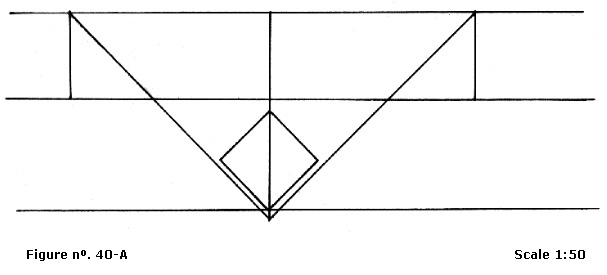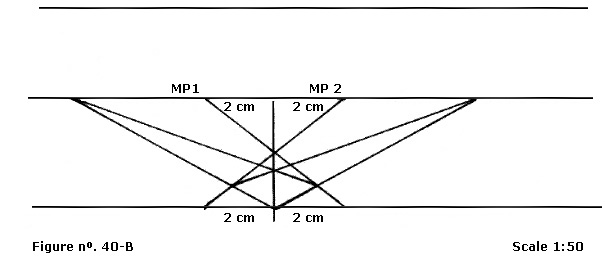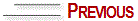# LOCATION OF THE MEASUREMENT POINTS (cont'd)

To project a plane in vertical position, as shown in the graph at the right side, first of all we have to find the measurement points 1 and 2 on the horizon line.

In this case, the view point coincides with the depth line and there is no intersection between them as in the projection of the slightly inclined vertical plane.

See in graph that the distances of vanishing points 1 and 2 are identical.To project the plane:-

Mark the vanishing points 1 and 2 and the depth point on the horizon line.

Also on the horizon line, mark the measurement points 1 and 2, (square measures) 2cm away from the view point. Place one point at the right and the other at the left.

Repeat the operation on the support line or measurement lines – 2cm at each side. Join the points forming an X, as shown at right.

At the crossing of the lines that run to vanishing points 1 and 2 with the lines that run from the support line to the measurement points 1 and 2 we will find the measurements of the horizontal plane.

See illustrative figure below: